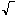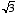index: click on a letter A B C D E F G H I J K L M N O P Q R S T U V W X Y Z A to Z index index: subject areas numbers & symbols sets, logic, proofs geometry algebra trigonometry advanced algebra & pre-calculus calculus advanced topics probability & statistics real world applications multimedia entrieswww.mathwords.com about mathwords website feedback

 Radicand The number under the(radical) symbol. That is, a number which is having its square root taken (or cube root, 4th root, 5th root, nth root, etc.). For example, 3 is the radicand in.     See also